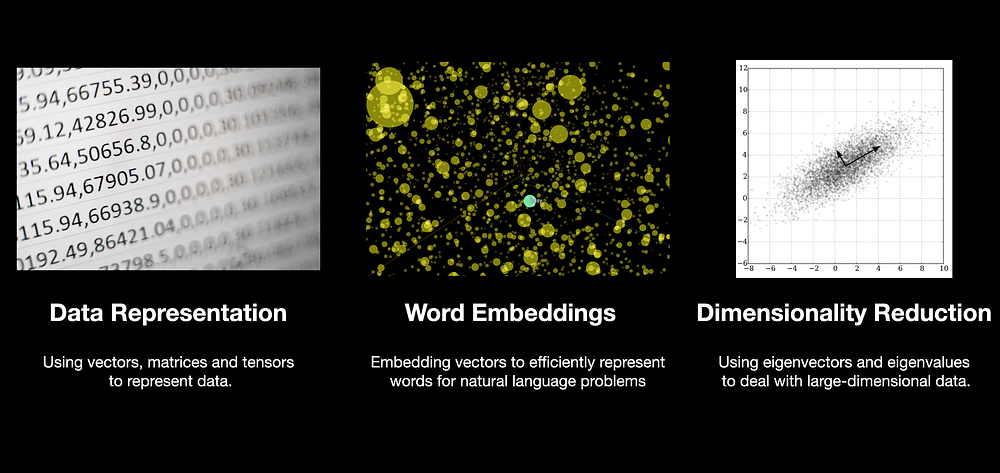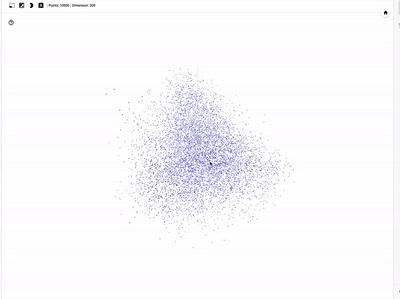By Harshit Tyagi, Data Science Instructor | Mentor | YouTuber

Machines or your computers only understand numbers and these numbers need to be represented and processed in a way that enables these machines to solve problems by learning from data instead of predefined instruction as in the case of programming.

All types of programming use mathematics at some level and machine learning is programming data to learn the function that best describes the data.

The problem(or process) of finding the best parameters of a function using data is called model training in ML.

Therefore, in a nutshell, machine learning is programming to optimize for the best possible solution and we need math to understand how that problem is solved.

The first step towards learning Math for ML is Linear algebra.

Linear Algebra is that mathematical foundation that solves the problem of representing data as well as computations in machine learning models.

It is the math of arrays — technically referred to as vectors, matrices, and tensors.

## Common Areas of Application — Linear Algebra in ActionIn the ML context, all major phases of developing a model have linear algebra running behind the scenes.

Important areas of application that are enabled by linear algebra are:

• data & learned model representation
• word embeddings
• dimensionality reduction

Data Representation — The fuel of ML models, a.k.a. data, needs to be converted into arrays before feeding into your models, the computations performed on these arrays include operations like matrix multiplication(dot product) which further returns the output that is also represented as a transformed matrix/tensor of numbers.https://projector.tensorflow.org/

Word embeddings — don’t worry about the terminology here, it is just about representing large-dimensional data(think of a huge number of variables in your data) with a smaller dimensional vector.

Natural Language Processing(NLP) deals with textual data. Dealing with text means comprehending the meaning of a large corpus of words and each word represents a different meaning which might be similar to another word, vector embeddings in linear algebra allow us to represent these words more efficiently.

Eigenvectors(SVD) — Finally, concepts like eigenvectors allow us to reduce the number of features or dimensions of the…